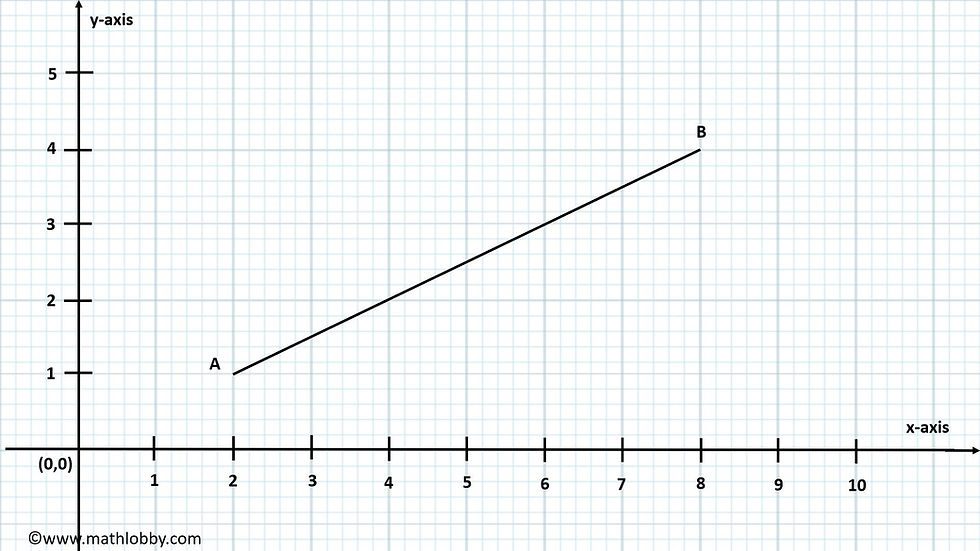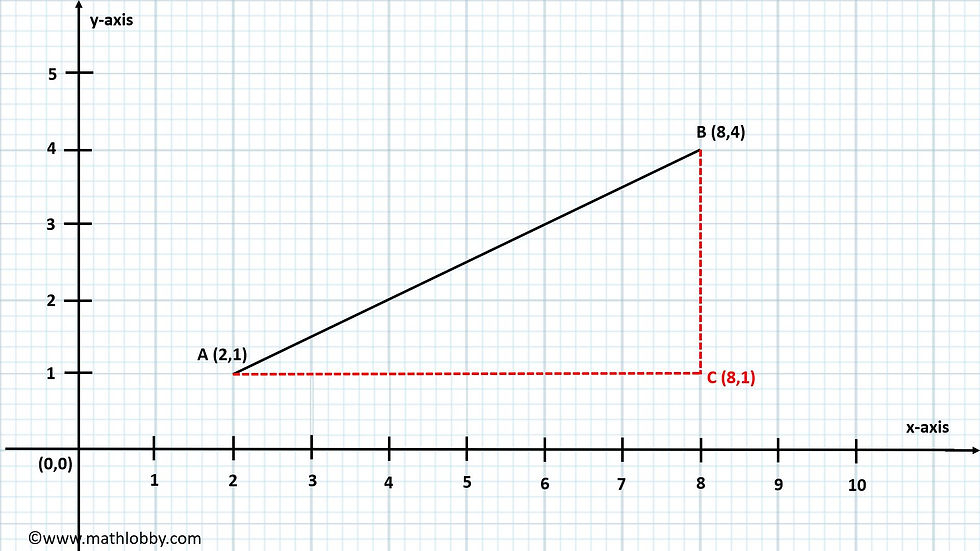top of page
Search

# How to find length of a line segment

Updated: Jun 22, 2021

Dear Secondary Math students, Math Lobby will be teaching you how to find length of a line segment on a graph (or just with the coordinates of its end points). By mathematics definition in layman terms, a line segment is part of a line with two distinct end points. Let’s begin!

### · How to find the length of a line segment given the coordinates of its end points

Given that we have a random line segment AB on a graph,To begin, we need to find the coordinates of the end-points of the line segment if we are not provided with it. From the graph above, we can clearly see that the coordinates of the end-points A and B to be (2,1) and (8,4) respectively.So now, how can we use this piece of information to find the length of the line segment? We might need a little help from a chapter we have previously covered: Pythagoras’ Theorem! (If you have not viewed our article on the Pythagoras’ Theorem, you can do so here!

How can the concept of Pythagoras’ Theorem aid us in solving this question in this case? Take a look at the end-points of the line, if we were to extrapolate the y-coordinate of A and x-coordinate of B, does it form something familiar?

Yes! You are right, it is a right-angled triangle! With this new-found information, we can apply the concept of Pythagoras’ Theorem to solve for the length of the line segment. Let’s see how it’s done:Through extrapolation, we can see that the y-coordinates of A and the x-coordinate of B intersects at the point (8,1) to give us a right-angled triangle. Hence, let’s name this intersection C. Now, let’s recap the formula we learnt back in Pythagoras’ Theorem: a² + b² = c², which in this case, a and b are essentially just AC and BC, and AB is essentially just the hypotenuse of the right-angled triangle!

How do we find the length of AC and BC then? Simple! To get the length of AC and BC, just simply subtract the x-coordinate of A by the x-coordinate of C and the y-coordinates of C by the y-coordinate of B respectively! This will give us a newly-derived formula that resembles the formula of Pythagoras’ Theorem, which we call it the distance formula.

### (Pythagoras’ Theorem solving for the hypotenuse, c = √( a2 + b2 ))

*Note: The order of subtraction (ie. whether to use point A to subtract point C or point C to subtract point A) does not matter, because 1) you will end up with the same values just with the exception of one being negative, 2) the squaring of the values will take out any negative signs and 3) we will always take the positive value because we know that length can never be negative!

Length of AB = [(8 -2)² + (4-1)²]

= 6.7082039325 units

~ 6.71 units (corrected to 3 s.f)

And that’s how you find the length of a line segment with the coordinates of the end-points!

Now, let’s try some examples:

1) Given that we have a line segment DE, where D (5, 12) and E (8, 10). Find the length of the line segment.

Answer: Length of DE = 3.61 units (corrected to 3 s.f) or √13 units

2) Given that we have a line segment FG, where F (-3, 15) and G (9, -4). Find the length of the line segment.

Answer: Length of FG = 22.5 units (corrected to 3 s.f) or √505 units

And that’s all we have for today, math students! Be sure to check out our other math articles as well on Math Lobby, and we hope that you understood the explanation and correct method to find the length of a line segment in a graph with the coordinates of the end-points.

Do leave us a like on Facebook, follow our Instagram and share this with all your fellow friends! As always: Work hard, stay motivated and we wish all students a successful and enjoyable journey with Math Lobby!

If you want more Secondary Math Tips, LIKE our Facebook page at www.facebook.com/mathlobbymotivation/

FOLLOW our Instagram page at https://www.instagram.com/mathlobbymotivation/